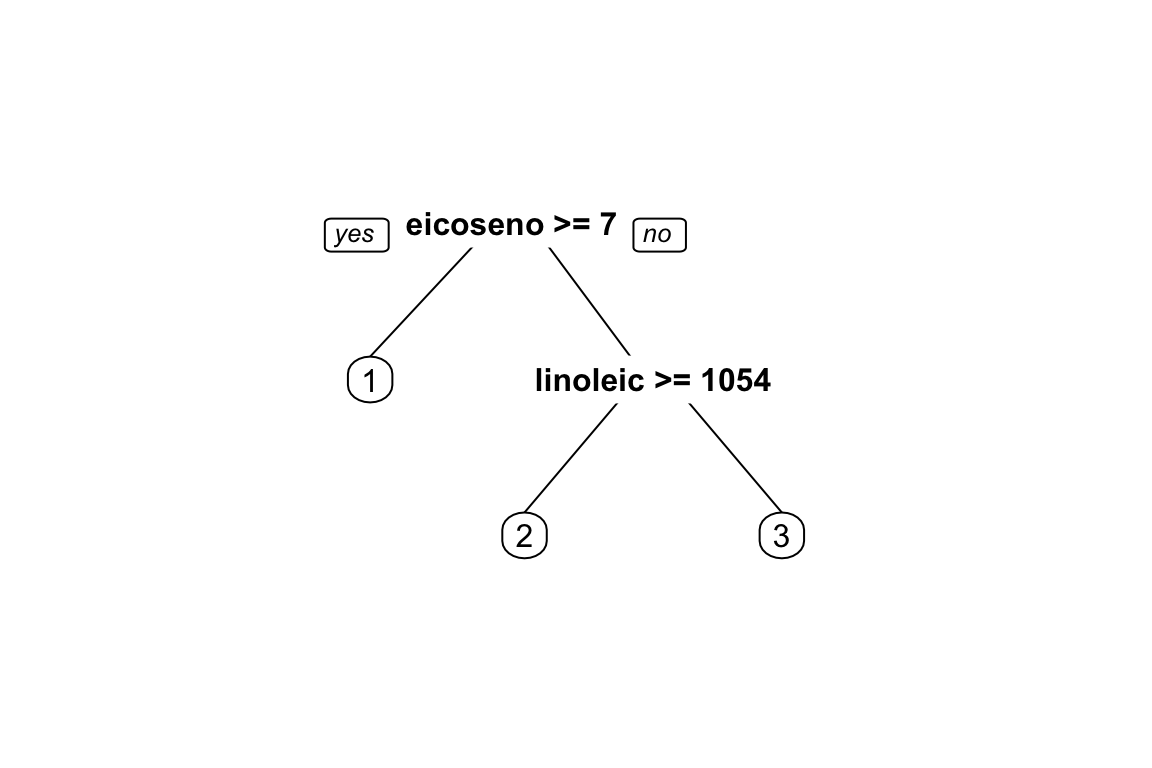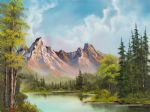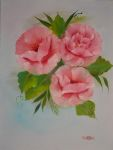# Objective

The objectives for this week are:

• Learn about fitting a forest model and use the diagnostics
• Compare the classification tree with a forest
• Understand the the idea of bagging to refine model fitting

# Class discussion

The focus this week is understanding how to determine the importance of variables in tree and forest models.

Question 1: In the following tree, what would you says is the order of importance of the variables for classifying region?Question 2: Read the explanation of how variable importance is calculated from the original developers of the algorithm (Leo Breiman and Adele Cutler), at https://www.stat.berkeley.edu/~breiman/RandomForests/cc_home.htm. Make a sketch to explain how this permutation approach can be used to measure variable importance.

# Practice

1. Fit the tree to olive oils, using a training split of 2/3, using only regions 2, 3, and the predictors linoleic and arachidic. Report the training and test error, and make a plot of the boundary.

2. Fit a random forest to the full data, using only linoleic and arachidic as predictors, report the out-of-bag error, and make a plot of the boundary.

3. Explain the difference between the single tree and random forest boundaries.

4. Fit the random forest again to the full set of variables, and compute the variable importance. Describe the order of importance of variables.

5. Create a new variable called linoarch that is $$0.377 \times linoleic + 0.926\times arachidic$$. Make a plot of this variable against arachidic. Fit the tree model to the same training data using this variable in addition to linoleic and arachidic. Check the test error too. Why doesn’t the tree use this new variable? It has a bigger difference between the two groups than linoleic?

6. Fit the random forest again to the full set of variables, including linoarch and compute the variable importance. Describe the order of importance of variables. Does the forest see the new variable?

7. This last question is see how random forests can be used in really tough problems. We use a forest to analyse the happy paintings by Bob Ross. This was the subject of the 538 post, “A Statistical Analysis of the Work of Bob Ross”.

We have taken the painting images from the sales site, read the images into R, and resized them all to be 20 by 20 pixels. Each painting has been classified into one of 8 classes based on the title of the painting. This is the data that you will work with.

It is provided in wide and long form. Long form is good for making pictures of the original painting, and the wide form is what you will need to use for fitting the classification models. In wide form, each row corresponds to one painting, and the rgb color values at each pixel are in each column. With a $$20\times20$$ image, this leads to $$400\times3=1200$$ columns.

Here are three of the original paintings in the collection, labelled as “scene”, “water”, “flowers”:1. How many paintings in the training data? Explain the difference between the long and the wide format of the data. What is the dimension of the data, used for the classification?
2. Build a random forest for the training data, for two classes, flowers and cold.
3. Predict the class of test set, report the error.
4. Which pixels are the most important for distinguishing these two types of paintings?